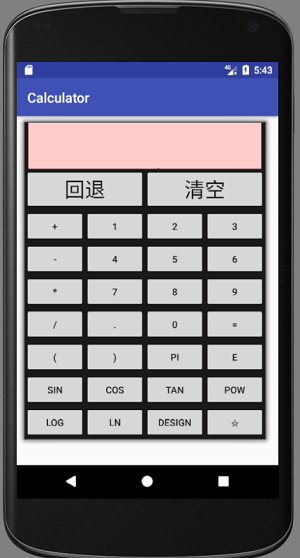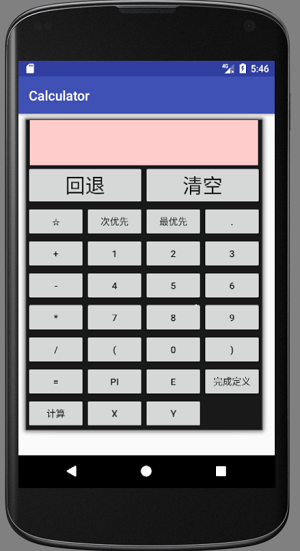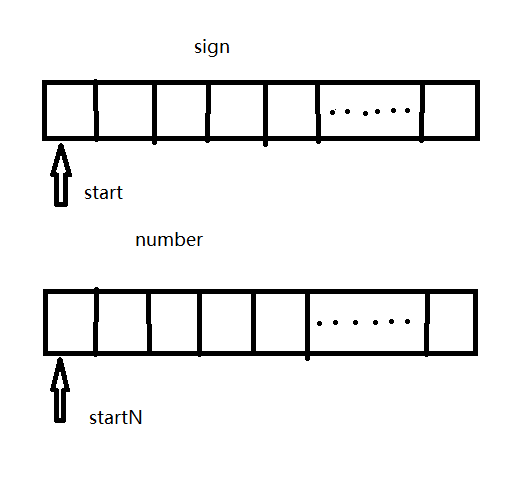# 安卓（java）计算器简单实现好了，那么今天我们来讲一下安卓计算器的简单实现，对于广大愁于编程的初学者大学生来说，自己制作一个计算器的作业会非常难，但是不要害怕，编程本来就很难，智商跟不上很正常，这不！博主所幸为您提供一个解决当务之急渠道，那么就正式开始今天的学习。

这里我们对于计算器界面的设计就略讲了，因为如果读者用android studio做的话界面布局是很简单的，只需用一个表格布局；而用eclipse做的话，就要采用java的swing技术来做界面，用的就是swing中的GridLayout布局管理器，不过出于创新，读者可以试着设计自己的布局方式，那么计算器的界面我就扯这么多了。

display：计算器的文本输入和显示框，每当用户输入表达式或删除表达式会在这个文本框中显示。

sign：承装符号的容器

number：承装数字的容器

private static TextView display;
private Button back,clear,plus,sub,mul,div,leftR,rightR,PI,e,point,equal,one,two,three,four,five,six,seven,eight,nine,zero,sin,cos,tan,pow,Log,ln,design,star;

static ArrayList<Character> sign=new ArrayList<Character>();
static ArrayList<Double> number=new ArrayList<Double>();

boolean isleftR=false;      //这个布尔值表示上一个被扫描的字符是不是左括号
int leftbound=0,rightbound; //leftbound表示要存入容器数据的左边界，rightbound则是有边界，比如3.56左边界指向3，有边界指向6，而单个字符则是左右边界相等
for(int i=0;i<processString.length();i++){          //扫描整个表达式
    if(processString.charAt(i)=='('){            //遇到左括号就将其加入sign，设置isleftR为true
isleftR=true;

}
else if(processString.charAt(i)==')'||processString.charAt(i)=='+'||processString.charAt(i)=='*'||processString.charAt(i)=='/'){                                                          //如果是右括号，加，乘，除就直接加入sign，重置isleftR
isleftR=false;

}else if(processString.charAt(i)=='-'&&!isleftR&&i!=0){R          //减号比较特殊，如果减号不是表达式的第一个符号，而且上一个字符不是左括号，则表示这个减号是运算的减号，否则是负号
isleftR=false;

}else if (processString.charAt(i)=='-'&&(isleftR||i==0)){  //负号的话我们要加进number的就不只是一个字符了，我们要加进负号连同它后面的数字，所以我们这里使用预先定义的左右边界，下面if的条件表示有边界选定的规则
if(i==0){
leftbound = i+1;
for (rightbound = leftbound; rightbound < processString.length(); rightbound++) {
if (processString.charAt(rightbound) == '(' || processString.charAt(rightbound) == ')' || processString.charAt(rightbound) == '+' || processString.charAt(rightbound) == '-' || processString.charAt(rightbound) == '*' || processString.charAt(rightbound) == '/') {
break;
}
}
if (rightbound == processString.length()) {         //如果有边界到了表达式的总长度处还没有确定，则视为最后一个字符为有边界
number.add(Double.parseDouble(processString.substring(leftbound-1, rightbound - 1) + processString.charAt(processString.length() - 1)));
}
isleftR = false;
i = rightbound - 1;

}else {
leftbound = i+1;
for (rightbound = leftbound; rightbound < processString.length(); rightbound++) {
if (processString.charAt(rightbound) == '(' || processString.charAt(rightbound) == ')' || processString.charAt(rightbound) == '+' || processString.charAt(rightbound) == '-' || processString.charAt(rightbound) == '*' || processString.charAt(rightbound) == '/') {
break;
}
}
if (rightbound == processString.length()) {
number.add(Double.parseDouble(processString.substring(leftbound-1, rightbound - 1) + processString.charAt(processString.length() - 1)));
}
isleftR = false;
i = rightbound - 1;
}
}else if((processString.charAt(i)>='0')&&(processString.charAt(i)<='9')){    //如果是数字的话，判断方式同负号一样
leftbound=i;
for(rightbound=leftbound;rightbound<processString.length();rightbound++){
if(processString.charAt(rightbound)=='('||processString.charAt(rightbound)==')'||processString.charAt(rightbound)=='+'||processString.charAt(rightbound)=='-'||processString.charAt(rightbound)=='*'||processString.charAt(rightbound)=='/'){
break;
}

}
if(rightbound==processString.length()){
}
isleftR=false;
i=rightbound-1;

}
else{        //输入其它非法符号则表达式错误
Toast.makeText(MainActivity,"计算表达式输入错误！",Toast.LENGTH_LONG).show();
}
}

以上是表达式的切分，到现在sign和number容器应该已经装满相应的数据了，然后我们就要以一定的顺序来计算这些数据了，也就是在sign和number这两个容器之间寻找一种规则，此处我们考虑先计算不带括号的表达式，再添加一个定位括号的算法进而完成带括号表达式的计算，所以下面介绍一下不带括号表达式的算法，例如： 输入3*5+2-4 ， 结果应该是13.

代码实现如下：参数解释：a,b为sign和number，length是sign长度，start和startN是指针用于操作容器，数据结构如上图所示
public static double compute_withoutR(ArrayList<Character> a,int start,int length,ArrayList<Double> b,int startN)
{
int beginvalue=start;
int count=0; //count计数已经算过的符号数
for(int i=start;beginvalue<start+length;beginvalue++)         //从头扫描符号容器，因为没有括号，所以先算乘除后算加减
{
if(a.get(i).equals('*')||a.get(i).equals('/')){              //这里我们只讲解乘号，因为后面的判定运算都相似
if(a.get(i).equals('*')) {
double temp = mul(b.get(startN+i-start),b.get(startN+i-start+1));  //读者不妨对应上面的数据结构看一下这规律，如果第i个符号是乘号，那么这个乘号所关系的两个乘数，其中一个必定是number的第startN+i-start个数字，另一个是第 startN+i-start+1个数字。
b.remove(startN+i-start);  //每计算完一次，都要把这次计算的两个数从number中移除，符号也要移除
b.remove(startN+i-start);

a.remove(i);
count++;
}else{
double temp = div(b.get(startN+i-start),b.get(startN+i-start+1));

b.remove(startN+i-start);
b.remove(startN+i-start);

a.remove(i);
count++;
}
}else {
i++;
}
}
beginvalue=start;
for(int i=start;beginvalue<start+length-count;beginvalue++){  //计算剩余符号
if(a.get(i).equals('+')||a.get(i).equals('-')){
if(a.get(i).equals('+')) {
double temp = Plus.plus(b.get(startN+i-start),b.get(startN+i-start+1));
b.remove(startN+i-start);
b.remove(startN+i-start);
a.remove(i);
}else{
double temp =Sub.sub(b.get(startN+i-start),b.get(startN+i-start+1));

b.remove(startN+i-start);
b.remove(startN+i-start);
a.remove(i);
}
}else{
System.out.println("basic运算错误！");
}
}

return b.get(startN);   //所有的符号计算完，number中的startN所指的数就是要返回的结果值
}目前为止，我们已经可以计算不带括号的表达式了，下面我要说的就是比较麻烦一点的带括号运算，其思路就是传入容器的一部分到上述的无括号运算函数中，这里一部分是指一个小括号里的内容，也就是说下面要介绍的是一个容器吞吐的函数，它的作用就是依照括号的顺序来计算表达式，先将表达式里所有的括号从右向左从里向外地计算完，剩下没有括号的表达式再做最后一次运算可得最终结果，因此搞明白如何匹配括号是下面这个函数的重点。代码实现：int leftRCount=0,leftRLastindex=-1,leftNindex=0,rightRindex=0; //leftRCount为左括号数，leftRLastindex指向容器中最后一个左括号，leftRindex指向最后一个左括号，rightRindex指向最后一个右括号。

for(int i=0;i<sign.size();i++){       //计数左括号数
if(sign.get(i).equals('(')){
leftRCount++;
}
}
if(leftRCount==0){       //如果没有左括号则表达式中无括号计算
display.setText(String.format("%.5f",BasicCompute.compute_withoutR(sign,0,sign.size(),number,0)));
sign.clear();
number.clear();
}else {
for (int loop = 0; loop < leftRCount; loop++) {       //有几个左括号我们就要计算几次括号内容

for (int i = 0; i < sign.size(); i++) {
if (sign.get(i).equals('(')) {
leftRLastindex = i;                    //确定leftRLastindex

}
}
for (int i = 0; i < sign.size(); i++) {
if (i < leftRLastindex && (sign.get(i).equals('+') || sign.get(i).equals('-') || sign.get(i).equals('*') || sign.get(i).equals('/'))) {
leftNindex++;                       //确定leftRindex
}
if (i > leftRLastindex && sign.get(i).equals(')')) {
rightRindex = i;                     //确定rightRindex
break;
}
}
double temp = BasicCompute.compute_withoutR(sign, leftRLastindex + 1, rightRindex - leftRLastindex - 1, number, leftNindex);
sign.remove(leftRLastindex);           //每计算一次都要把该次的小括号从sign中移除
sign.remove(leftRLastindex);
leftNindex = 0;
}
temp=String.format("%.5f", BasicCompute.compute_withoutR(sign, 0, sign.size(), number, 0));            //本次运算是括号都计算后的最后一次无括号运算，是括号运算的最后一步，相当于上面的无括号运算。
display.setText(String.format("%.5f", BasicCompute.compute_withoutR(sign, 0, sign.size(), number, 0)));
sign.clear();
number.clear();

}
return temp; //temp为最终的计算值到现在为止计算器的基本内容也是核心内容就讲解完毕了，剩下的其它科学运算建议采用隔离法，意思是在输入表达式的时候就让特殊的计算进行完毕，让后其结果值返回至计算器显示文本中，作为表达式的一部分，而不必所有的运算都要在输入表达式之后统一处理，另外还有精度、表达式输入正确检测、错误提示等细节功能需要依据个人风格来完成。最后我们来总结一下这个算法的优点与缺点：

05-074万+09-146568
04-143445
04-01645
09-091万+
03-171万+
07-284万+
03-20
09-20